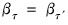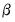qrslope
Perform Wald test of equality of slope coefficients across multiple quantile regression estimates. The equality test restrictions are of the form:for the slope coefficients.
Syntax
eq_name.qrslope(options) [arg] [@coefs coeflist]
where arg is a optional list containing the quantile values (specified using numbers, scalar objects, or vectors) for which you wish to compute estimates, and optionally the @coefs keyword followed by a coeflist of the subset of coefficients to display.
If arg is not specified, EViews will perform tests for the existing equation and coefficients for equations estimated at a set of equally spaced quantiles as specified by the “n=” option. If “n=” is not specified, the default is to display results for the quartiles (.25, .75).
If arg is specified, EViews will perform results for the original equation along with tests including coefficients for equations estimated at the specified quantiles.
If a coeflist is not provided, all of the slope coefficients will be employed in the test.
You may specify a maximum of 1000 total coefficients (number of coefficients in the equation specification times the number of quantiles) and a maximum of 500 quantiles in the test.
All estimation will be performed using the settings from the original equation.
Options

 n=arg (default=4) Number of quantiles for process estimates. quantout=name Save vector containing test quantile values. coefout=name Save matrix containing test coefficient estimates. Each column of the matrix corresponds to a different quantile matching the corresponding quantile in “quantout=”.To match the covariance matrix given in “covout=” you should take the @vec of the coefficient matrix. covout=name Save symmetric matrix containing covariance matrix for the vector set of coefficient estimates. prompt Force the dialog to appear from within a program. p Print output from the test.
Examples
equation eq1.qreg log(y) c log(x)
eq1.qrslope
estimates a quantile (median) regression of LOG(Y) on a constant and LOG(X), and tests for the equality of the coefficients of LOG(X) for all three of the quartiles.
Similarly,
equation eq1.qreg(quant=.4) log(y) c log(x)
eq1.qrslope(coefcout=cout)
tests for equality of the LOG(X) coefficient estimated at {.25, .4, .5, .75}, and saves the coefficient matrix to COUT. Both
eq1.qrslope(coefout=count, n=10)
eq1.qrslope(coefout=cout) .1 .2 .3 .4 .5 .6 .7 .8 .9
perform the Wald test for equality of the slope coefficient across all of the deciles, as does the equivalent
vector v1(9)
v1.fill .1,.2,.3,.4,.5,.6,.7,.8,.9
eq1.qrslope v1
Cross-references
See “Slope Equality Test” for a discussion of the slope equality test.